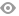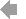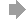Basic HTML VersionView Full Version
Page 40 - Course Selection Guide 2021-2022
P. 40

CONCEPTS IN INTERMEDIATE ALGEBRA (530)
Year 1 Credit Grades 11, 12 Req: Geometry and Department approval
This course is part of the Alternate Sequence for students completing Geometry 521A or who consistently have had below average grades or lower in Algebra I and Geometry. The topics covered are the same as in the Algebra II/Trig course, except for the Trigonometry portion of the course. Topics covered include: real numbers, equations and inequalities, graphing, systems of sentences, relations and functions, polynomials, rational expressions, radicals, quadratic functions, complex numbers, quadratic equations, exponential and logarithmic functions, and using probability distributions.
MATH TOPICS (541)
Year 1 Credit Grade 11, 12 Req: Concepts in Intermediate Algebra or Algebra II/Trig; grade 11 requires Department approval
This is a less rigorous course than Math Analysis and contains similar topics. It is for fourth year students and is designed to prepare them for taking a college math course and is not for science/engineering students. The approach and pace of the course are geared for the students who have had average or less than average grades in Math during their first three years of math. Topics include trigonometry, logarithms, vectors, matrices, sequences and series, complex numbers, probability and statistics. A graphing calculator is required.
MATH ANALYSIS (542)
Year 1 Credit Grades 11, 12 Req: Honors Algebra II/Trig or Algebra II/Trig.
This rigorous course in math is for students with a good math background; it is the standard course for the fourth year of math. Topics covered: sequences and series, conics, trigonometric functions, complex numbers, limits, polar coordinates, vectors, analytical geometry, matrices, and exponential and logarithmic functions. A graphing calculator is required.
HONORS MATH ANALYSIS/INTRO. TO CALCULUS (576)
Year 1 Credit Grades 11, 12 Req: Honors Geometry/Algebra II or Honors Algebra II/Trig; Algebra II/Trig with department approval.
This honors course is for the exceptional math student. Most students are juniors who plan to take an Advanced Placement/Honors Calculus course in their Senior year. It is, however, open to qualified seniors. The first three quarters cover Math Analysis topics at an accelerated pace. The emphasis is on functions, trigonometry, and skills necessary for calculus. Students will also learn test-taking skills for the PSAT/NMSQT. The fourth quarter will cover limits, and topics from calculus, including derivatives, curve sketching, and applications of differentiation. A graphing calculator is required. Because of the rigors of this course, students are expected to maintain at least a C average each semester in order to maintain Honors status.
CALCULUS (577)
Year 1 Credit Grade 12 Req: Math Analysis and Department approval
This rigorous course in mathematics is intended for students with a strong mathematics background. The first semester includes work on derivatives, integrals, and the application of differentiation formulas. The second semester will cover transcendental functions, methods of integration, hyperbolic functions and polar coordinates. Other topics in integral and differential calculus will be offered to meet the needs of the students. A graphing calculator is required.
3938   39   40   41   42